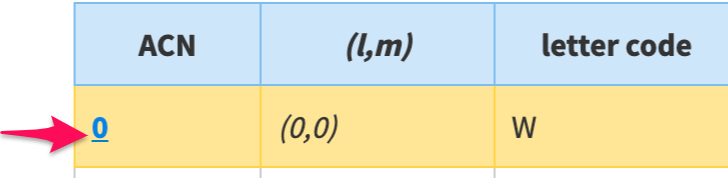# Ambisonic Channels Sequence

Index page

October 2008: The proposal documented here has engendered wide support. It is though intended for future ambisonic files (etc.).
Pre-2009 files typically used a channel sequence of WXYZRSTUVKLMNOPQ (the table below can be used as a concordance), and FuMa channel weightings (conversion factors are on the pages for channels 0 to 15).

The current most favoured proposal for ambisonic channel sequence seems to be to define an ambisonic channel number (ACN), such that:
`ACN = l * ( l + 1 ) + m`
(For the definition of l and m see the Glossary.)Click on an ACN (blue-number) for full details about that channel.

Ambisonics Channel number and formulas
ACN
(l,m)
letter code
(0,0)
W
(1,-1)
Y
(1,0)
Z
(1,1)
X
(2,-2)
V
(2,-1)
T
(2,0)
R
(2,1)
S
(2,2)
U
(3,-3)
Q
(3,-2)
O
(3,-1)
M
(3,0)
K
(3,1)
L
(3,2)
N
(3,3)
P
(4,-4)

(4,-3)

(4,-2)

(4,-1)

(4,0)

(4,1)

(4,2)

(4,3)

(4,4)

(5,-5)

(5,-4)

(5,-3)

(5,-2)

(5,-1)

(5,0)

(5,1)

(5,2)

(5,3)

(5,4)

(5,5)

(6,-6)

(6,-5)

(6,-4)

(6,-3)

(6,-2)

(6,-1)

(6,0)

(6,1)

(6,2)

(6,3)

(6,4)

(6,5)

(6,6)

(7,-7)

(7,-6)

(7,-5)

(7,-4)

(7,-3)

(7,-2)

(7,-1)

(7,0)

(7,1)

(7,2)

(7,3)

(7,4)

(7,5)

(7,6)

(7,7)

(8,-8)

(8,-7)

(8,-6)

(8,-5)

(8,-4)

(8,-3)

(8,-2)

(8,-1)

(8,0)

(8,1)

(8,2)

(8,3)

(8,4)

(8,5)

(8,6)

(8,7)

(8,8)

(9,-9)

(9,-8)

(9,-7)

(9,-6)

(9,-5)

(9,-4)

(9,-3)

(9,-2)

(9,-1)

(9,0)

(9,1)

(9,2)

(9,3)

(9,4)

(9,5)

(9,6)

(9,7)

(9,8)

(9,9)

(10,-10)

(10,-9)

(10,-8)

(10,-7)

(10,-6)

(10,-5)

(10,-4)

(10,-3)

(10,-2)

(10,-1)

(10,0)

(10,1)

(10,2)

(10,3)

(10,4)

(10,5)

(10,6)

(10,7)

(10,8)

(10,9)

(10,10)

(11,-11)

(11,-10)

(11,-9)

(11,-8)

(11,-7)

(11,-6)

(11,-5)

(11,-4)

(11,-3)

(11,-2)

(11,-1)

(11,0)

(11,1)

(11,2)

(11,3)

(11,4)

(11,5)

(11,6)

(11,7)

(11,8)

(11,9)

(11,10)

(11,11)

Index page